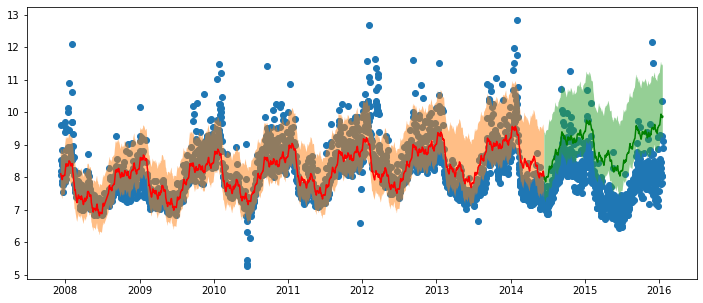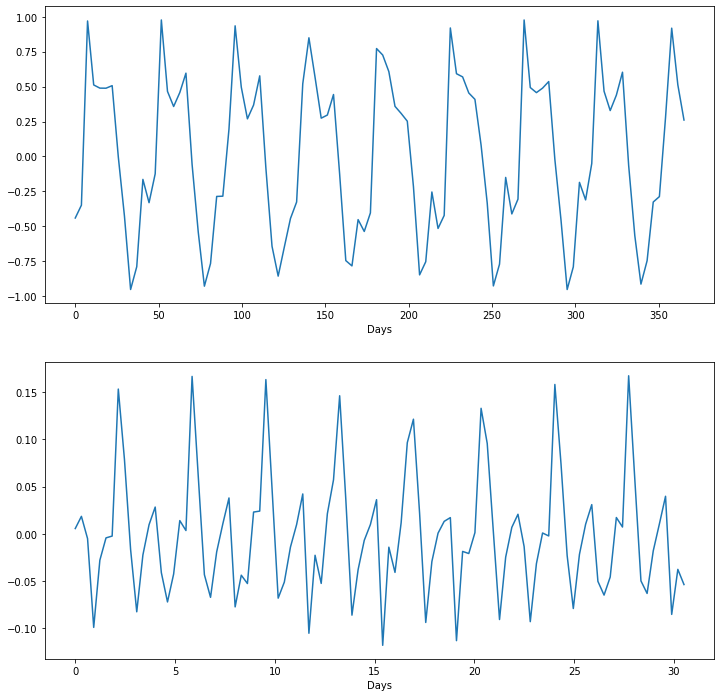FB Prophet + Fastai + pyTorch.

This is an alternative implementation of prophet which uses quantile regression instead of MCMC sampling. It provides the following benefits over prophet:

• GPU usage.
• Strict(er) upper and lower bounds.
• Can add any other set of features to the time series.

The time series is implemented as follows: \begin{aligned} y &= b(T(t) + S(t) + F(x)|l,u) \\ T(t) &= mt + a \\ S(t) &= \sum_{n=1}^N\left(a_n \cos\left(\frac{2\pi nt}{P}\right) + b_n \sin\left(\frac{2\pi nt}{P}\right)\right) \\ F(x) &= w^T x\\ b(y|l,u) &= \begin{cases} l \quad \text{if } y < l \\ y \quad \text{if } l < y < u \\ u \quad \text{if } y > u \end{cases} \end{aligned}

where $T(t)$ is the trend line, $S(t)$ are the seasonal components composed of a fourier sum, $F(x)$ is a linear function which weights features that is not related to time.

The task is therefore to find the parameters $a, m, \cup_n a_n, \cup_n b_n, w$ that minimises a loss function $l(\hat{y}, y)$. The default is set to minimise $l1$ loss $\frac{1}{N}\sum_{i=1}^N |y_i - \hat{y_i}|$ so that the reliance on outliers is minimised. By default we also calculate the 5th and 95th quantile by minimising the tilted loss function. The quantile functions are calculated as: \begin{aligned} y_5 &= b(\hat{y} - (m_5 t + a_5)|l,u) \\ y_{95} &= b(\hat{y} + (m_{95} t + a_{95})|l,u) \end{aligned}

## Install¶

pip install profetorch

## ProFeTorch Training¶

model_params = {'y_n':10, 'm_n':7, 'l':0, 'h': train_df['y'].max() * 2}
model = Model(model_args=model_params, epochs=30, alpha=1e-2)
model.fit(train_df)

/opt/miniconda3/lib/python3.7/site-packages/pandas/core/frame.py:4117: SettingWithCopyWarning:
A value is trying to be set on a copy of a slice from a DataFrame

See the caveats in the documentation: http://pandas.pydata.org/pandas-docs/stable/user_guide/indexing.html#returning-a-view-versus-a-copy
errors=errors,

Epoch 30/30 Training Loss: 0.3680, Validation Loss: 0.5957

y_pred = model.predict(df)
plt.figure(figsize=(12,5))
plt.scatter(df['ds'], df['y'], label='Data')
plt.plot(train_df['ds'], y_pred[:train_len,1], c='r', label='Train Set')
plt.fill_between(train_df['ds'], y_pred[:train_len,0], y_pred[:train_len,2], alpha=0.5)
plt.plot(test_df['ds'], y_pred[train_len:,1], c='g', label='Test Set')
plt.fill_between(test_df['ds'], y_pred[train_len:,0], y_pred[train_len:,2], alpha=0.5)
plt.show()model.plot_components()list(model.named_parameters())

[('model.models.0.trend.init_layer.weight', Parameter containing:
('model.models.0.trend.init_layer.bias', Parameter containing:
('model.models.1.trend.init_layer.weight', Parameter containing:
('model.models.1.trend.init_layer.bias', Parameter containing:
('model.models.1.seasonal.yearly.linear.weight', Parameter containing:
tensor([[-0.2984,  0.0334, -0.1572, -0.0445,  0.0025,  0.0630,  0.0033, -0.0534,
0.0085,  0.0018, -0.6378, -0.1152,  0.1973,  0.1215,  0.0867, -0.0102,
('model.models.1.seasonal.monthly.linear.weight', Parameter containing:
tensor([[-0.0315,  0.0295,  0.0279, -0.0243, -0.0060, -0.0025,  0.0127,  0.0304,
-0.0582,  0.0004,  0.0020,  0.0055,  0.0186, -0.0176]],
tensor([0.9838], requires_grad=True))]